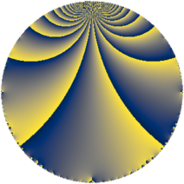# Properties

 Label 138.2.dLevel $138$ Weight $2$ Character orbit 138.d Rep. character $\chi_{138}(137,\cdot)$ Character field $\Q$ Dimension $8$ Newform subspaces $1$ Sturm bound $48$ Trace bound $0$

# Related objects

## Defining parameters

 Level: $$N$$ $$=$$ $$138 = 2 \cdot 3 \cdot 23$$ Weight: $$k$$ $$=$$ $$2$$ Character orbit: $$[\chi]$$ $$=$$ 138.d (of order $$2$$ and degree $$1$$) Character conductor: $$\operatorname{cond}(\chi)$$ $$=$$ $$69$$ Character field: $$\Q$$ Newform subspaces: $$1$$ Sturm bound: $$48$$ Trace bound: $$0$$

## Dimensions

The following table gives the dimensions of various subspaces of $$M_{2}(138, [\chi])$$.

Total New Old
Modular forms 28 8 20
Cusp forms 20 8 12
Eisenstein series 8 0 8

## Trace form

 $$8 q - 4 q^{3} - 8 q^{4} - 4 q^{6} + O(q^{10})$$ $$8 q - 4 q^{3} - 8 q^{4} - 4 q^{6} + 4 q^{12} - 8 q^{13} + 8 q^{16} - 16 q^{18} + 4 q^{24} + 48 q^{25} - 28 q^{27} + 16 q^{31} + 24 q^{39} + 16 q^{46} - 4 q^{48} - 56 q^{49} + 8 q^{52} + 28 q^{54} - 64 q^{55} + 8 q^{58} - 8 q^{64} + 28 q^{69} - 24 q^{70} + 16 q^{72} - 8 q^{73} - 4 q^{75} - 16 q^{78} + 8 q^{81} + 24 q^{85} - 56 q^{87} - 8 q^{93} + 56 q^{94} - 4 q^{96} + O(q^{100})$$

## Decomposition of $$S_{2}^{\mathrm{new}}(138, [\chi])$$ into newform subspaces

Label Dim $A$ Field CM Traces $q$-expansion
$a_{2}$ $a_{3}$ $a_{5}$ $a_{7}$
138.2.d.a $8$ $1.102$ 8.0.$$\cdots$$.3 None $$0$$ $$-4$$ $$0$$ $$0$$ $$q+\beta _{1}q^{2}+(\beta _{1}+\beta _{7})q^{3}-q^{4}+\beta _{3}q^{5}+\cdots$$

## Decomposition of $$S_{2}^{\mathrm{old}}(138, [\chi])$$ into lower level spaces

$$S_{2}^{\mathrm{old}}(138, [\chi]) \cong$$ $$S_{2}^{\mathrm{new}}(69, [\chi])$$$$^{\oplus 2}$$